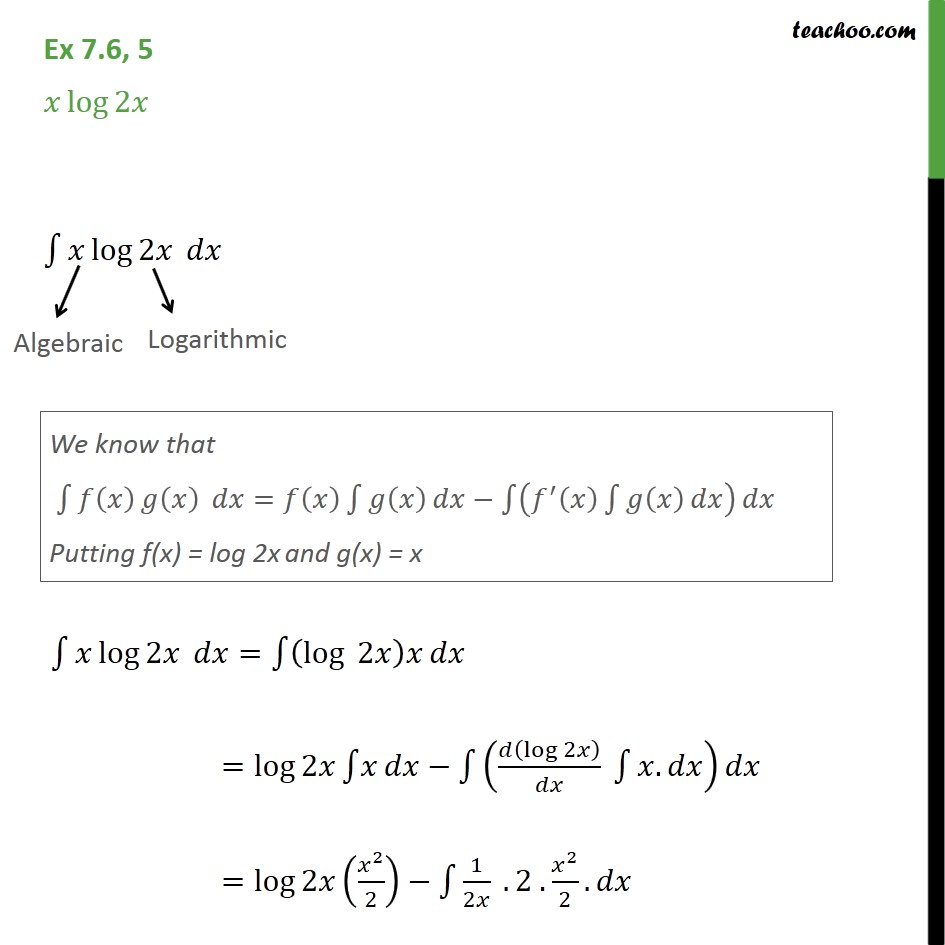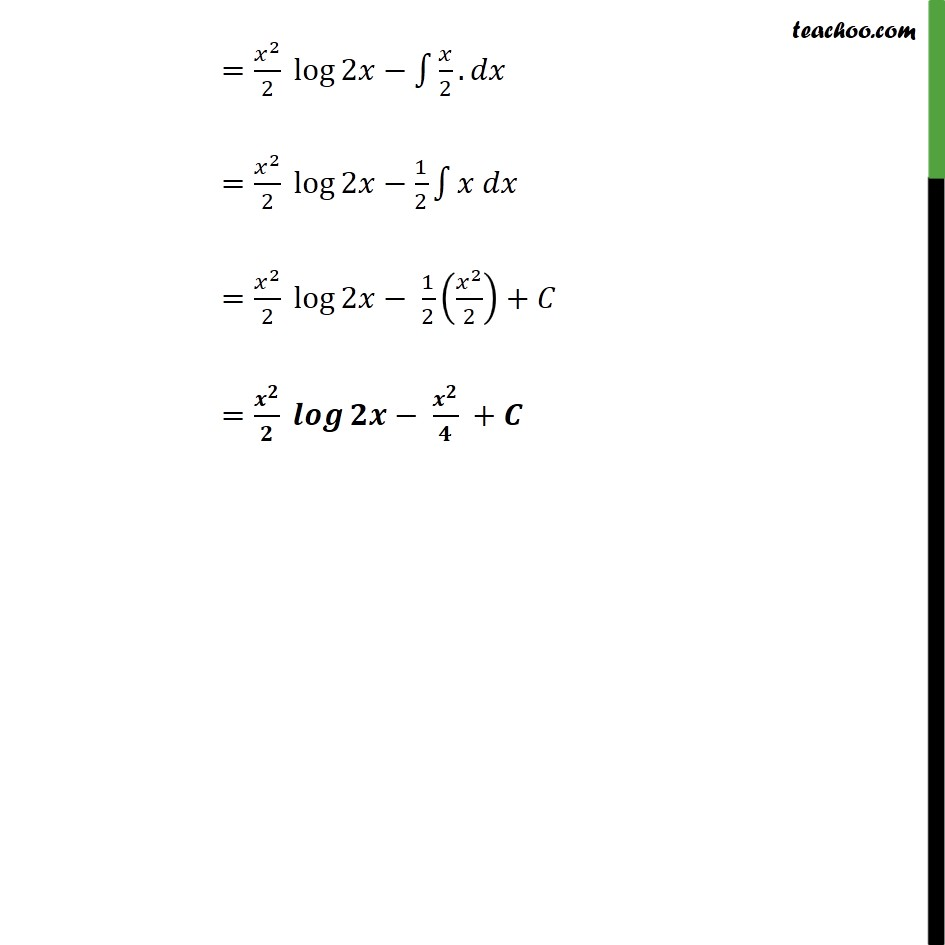1. Chapter 7 Class 12 Integrals (Term 2)
2. Serial order wise
3. Ex 7.6

Transcript

Ex 7.6, 5 𝑥 log⁡2𝑥 Integrating the function 𝑤.𝑟.𝑡.𝑥 ∫1▒〖𝑥 log⁡2𝑥 〗 𝑑𝑥 Hence, we take First function :- 𝑓(𝑥)=log⁡(2𝑥) Second function :- g(𝑥)=𝑥 ∫1▒〖𝑥 log⁡𝑥 〗 𝑑𝑥=∫1▒(log⁡𝑥 )𝑥 𝑑𝑥 =log⁡(2𝑥) ∫1▒𝑥 𝑑𝑥−∫1▒(𝑑(log⁡2𝑥 )/𝑑𝑥 ∫1▒〖𝑥.𝑑𝑥〗)𝑑𝑥 =log⁡(2𝑥) (𝑥^2/2)−∫1▒〖1/2𝑥 . 2 .𝑥^2/2. 𝑑𝑥〗 =〖𝑥^2/2 log〗⁡(2𝑥)−∫1▒〖𝑥/2. 𝑑𝑥〗 =〖𝑥^2/2 log〗⁡(2𝑥)−1/2 ∫1▒〖𝑥 𝑑𝑥〗 =〖𝑥^2/2 log〗⁡(𝑥)− 1/2 (𝑥^2/2)+𝐶 =〖𝑥^2/2 log〗⁡(2𝑥)− 𝑥^2/4 +𝐶